# Learn Validating User Input in Linux Shell Scripting

0
11379When a script gets one or more inputs from the user, most of the time it expects the following:

• A specific number inputs.
• Inputs of specific type or format.

If the user provides the wrong number of inputs, or inputs of incorrect type or format, the script may generate an error, and/or behave oddly (and of course, you don’t like to see either of these results).

For this reason, it is strongly recommended to spend some extra time writing code that validate user’s input.

* * * * * *

The Division by Zero Problem
Consider the calculator example. If the user enters 0 as the divisor, the division operation will be something over 0 (which we know from mathematics that it is an illegal operation). If no validation is place, the bash interpreter will try to execute the division operation, and … will report a division by zero error.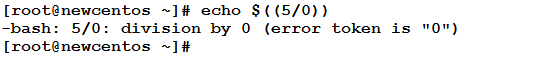The solution is to validate the user’s input to assure the divisor is not 0. Otherwise, a friendly error message is printed to the user.
Consider the following script that performs the required validation: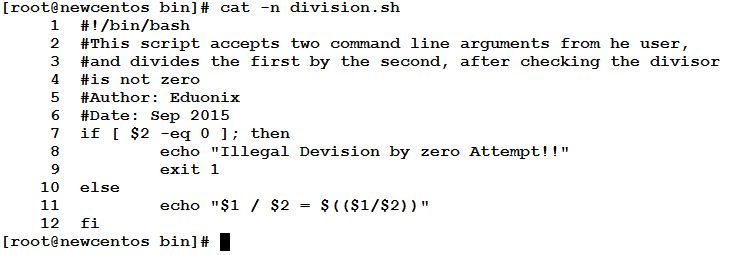How this will execute? Let’s see: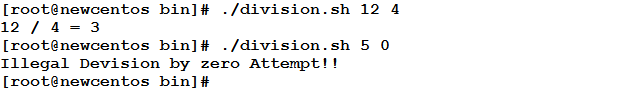The Factorial Example
Back again to the factorial example. In this time, we are going to write the script with input validation. Factorial is calculated for positive integers. So, we have to make sure the user’s input is a positive integer.

Consider the following script: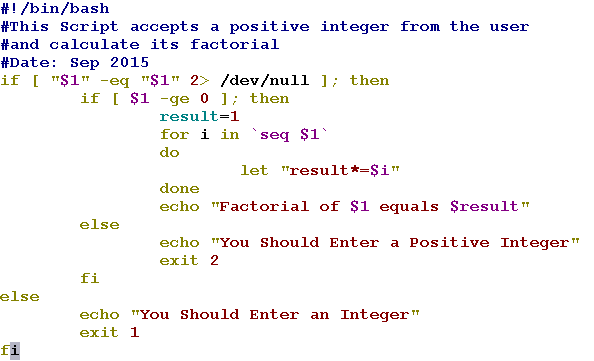Let’s see it in action: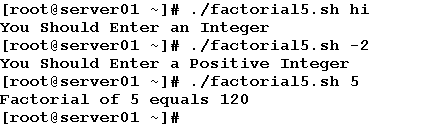Now, to the explanation.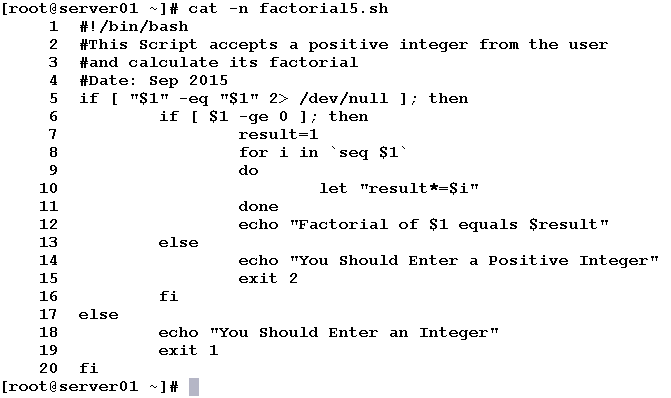• Line 5: checks if the provided command-line argument is a number, and redirects the error (that would be fired if the argument is non-numeric) to /dev/null. If found to be numeric, Line 6 will be executed.
• Line 6: again, the “numeric” argument is checked if it is greater than or equal to 0. If found to be non-negative, Lines from 7 to 12 will be executed.
• The result variable is initialized to 1.
• A for loop with loop variable i is defined to iterate on values in the range from 1 to the provided integer \$1.
•  Lines 9 and 11: the loop body delimiters.
• Line 10: the loop body. The current value of the loop variable is multiplied by the value of result. The multiplication result is stored in the variable result.
• Line 12: prints the result to the user.
• Line 13: Else (if the argument was found to be negative)
• Line 14: a message is printed to the user that he should enter a positive integer.
• Line 15: the program is exited with code 2
• Line 16: the closing fi for the inner if statement.
• Line 17: Else (if the input found to be non-numeric)
• Line 18: prints a message to the user that he should enter a numeric value.
• Line 19: exits the program with exit code 1.
• Line 20: the closing fi for the outer if statement.

So simple and logical, isn’t it?!

* * * * * *

Validating the Correct Number of Inputs
What if your script is expecting two arguments and the user enters only one, or didn’t provide any input at all?
Again, there should be a validation step.Example
In the Factorial example, the script is expecting one (and only one) numeric argument. In the previous example, we have learned how to verify the input is really a number, and non-negative. Now, in this modified version, we are going to check the number of arguments to verify the script will get the single argument it expects.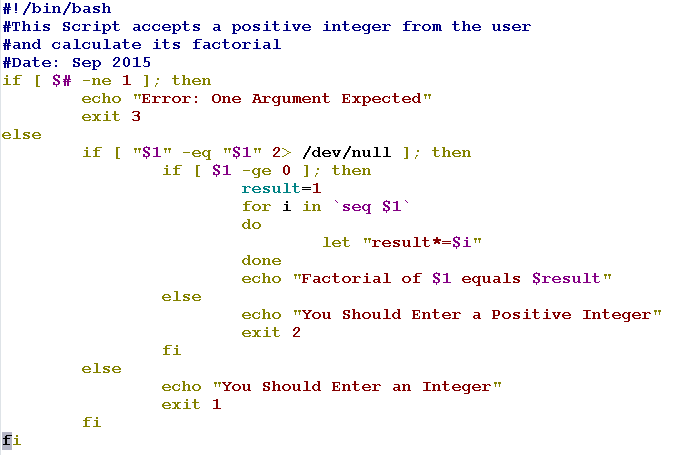Now, let’s see what we will get if we enter the wrong number of arguments.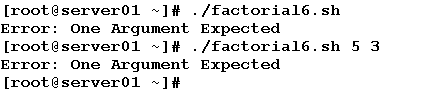In this modified version, we have made use of the \$# special variable. We know from an earlier article (Command-Line Arguments) that this variable represents the number of command-line arguments passed to an executed script. The idea is so simple, an if condition checks whether the \$# variable is equal to the expected number of arguments (which is one in this example). If not, an error message is printed to the user, and the script exits with an exit code 3. Else, the other checks are made as in the previous example.

Printing Usage Messages
Usage Messages are notifications to the user to help him know the correct syntax of the program. These messages are usually printed to the user either when he uses the –help option, or when he attempts to execute the program using the wrong syntax.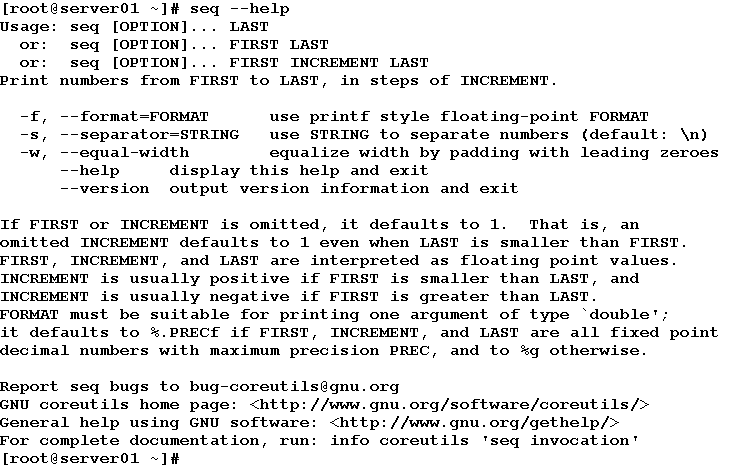In general, it is a best practice to provide users of your scripts with the necessary help messages that illustrate:

• The purpose of the script.
• The expected arguments and their types.
• The available options (if any).

Example
Back to the division example. Now, we are going to write a modified version that displays a usage message when the user executes the script with –help option, or if he enters incorrect number of arguments.

Consider the following code: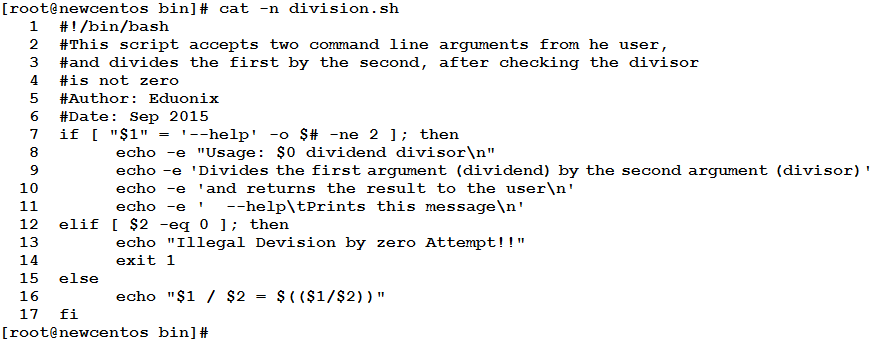Let’s see the modified version in action: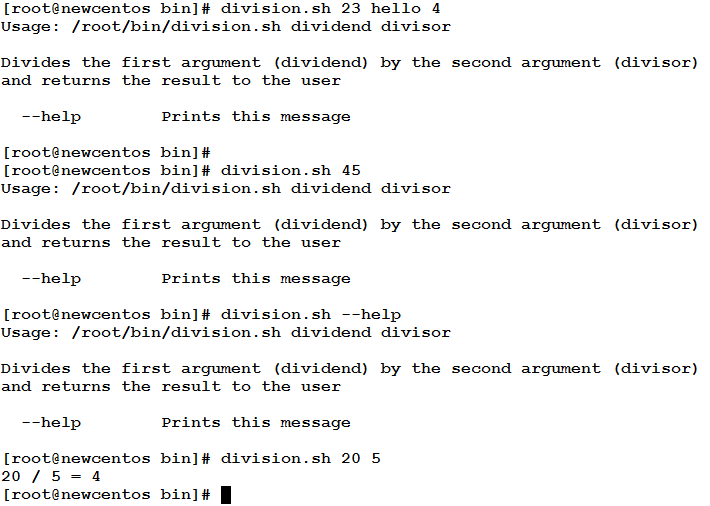As planned, the script will print the usage message if the user enters wrong number of input, or used the –help option. To achieve this, an if statement is used to check for these conditions.

* * * * * *

In this article, we have talked about User Input Validation. Validating user input is a best practice to eliminate a big source of execution errors and bugs. We have also learned how to print usage messages to tell users the purpose of the program, the expected arguments, and the available options.

See you in the next article.